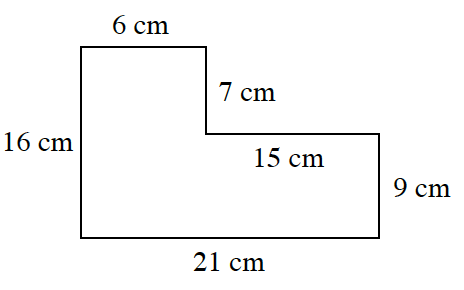### Home > INT2 > Chapter 1 > Lesson 1.1.1 > Problem1-8

1-8.

Angela has a rectangular piece of paper and cuts a rectangle out of a corner as shown at right. What are the area and perimeter of the resulting shape?

Perimeter is the distance around the exterior of a figure.

Area is the number of 'squares' needed to cover the interior of a figure.

Divide this shape into smaller rectangles to find the area.

$6+7+15+9+21+16=74 \text{ cm}$